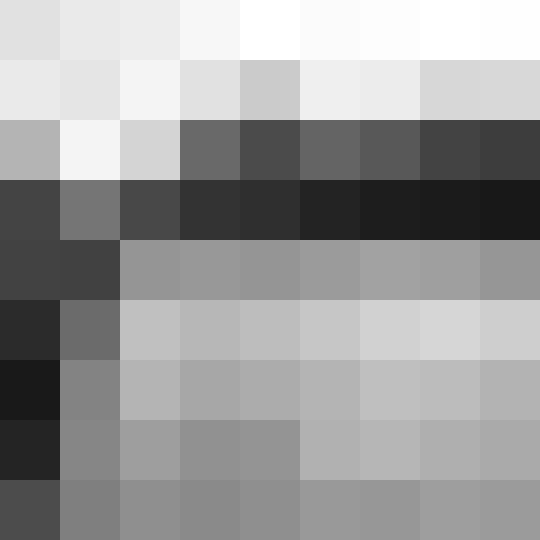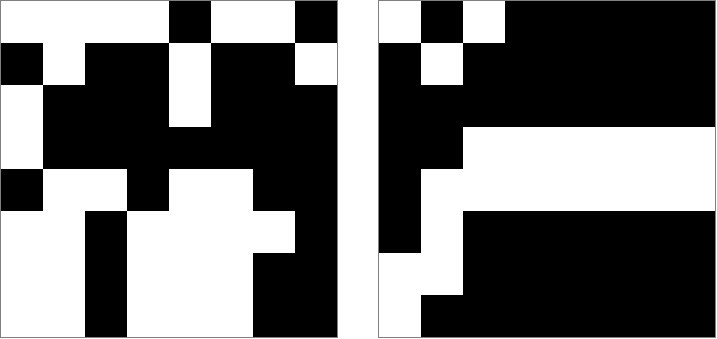# Duplicate image detection with perceptual hashing in Python

March 2017

Recently we implemented a duplicate image detector to avoid importing dupes into Jetsetter’s large image store. To achieve this, we wrote a Python implementation of the dHash perceptual hash algorithm and the nifty BK-tree data structure.

Jetsetter has hundreds of thousands of high-resolution travel photos, and we’re adding lots more every day. The problem is, these come from a variety of sources and are uploaded in a semi-automated way, so there are often duplicates or almost-identical photos that sneak in. And we don’t want our photo search page filled with dupes.

So we decided to automate the task of filtering out duplicates using a perceptual hashing algorithm. A perceptual hash is like a regular hash in that it is a smaller, compare-able fingerprint of a much larger piece of data. However, unlike a typical hashing algorithm, the idea with a perceptual hash is that the perceptual hashes are “close” (or equal) if the original images are close.

## Difference hash (dHash)

We use a perceptual image hash called dHash (“difference hash”), which was developed by Neal Krawetz in his work on photo forensics. It’s a very simple but surprisingly effective algorithm that involves the following steps (to produce a 128-bit hash value):

• Convert the image to grayscale
• Downsize to a 9x9 square of gray values (or 17x17 for a larger, 512-bit hash)
• Calculate the “row hash”: for each row, move from left to right, and output a 1 bit if the next gray value is greater than or equal to the previous one, or a 0 bit if it’s less (each 9-pixel row produces 8 bits of output)
• Calculate the “column hash”: same as above, but for each column, move top to bottom
• Concatenate the two 64-bit values together to get the final 128-bit hash

dHash is great because it’s fairly accurate, and very simple to understand and implement. It’s also fast to calculate (Python is not very fast at bit twiddling, but all the hard work of converting to grayscale and downsizing is done by a C library: ImageMagick+wand or PIL).

Here’s what this process looks like visually. Starting with the original image:Grayscale and down-sized to 9x9 (but then magnified for viewing):And the 8x8 row and column hashes, again magnified (black = 0 bit, white = 1 bit):The core of the dHash code is as simple as a couple of nested `for` loops:

``````def dhash_row_col(image, size=8):
width = size + 1
grays = get_grays(image, width, width)

row_hash = 0
col_hash = 0
for y in range(size):
for x in range(size):
offset = y * width + x
row_bit = grays[offset] < grays[offset + 1]
row_hash = row_hash << 1 | row_bit

col_bit = grays[offset] < grays[offset + width]
col_hash = col_hash << 1 | col_bit

return (row_hash, col_hash)
``````

It’s a simple enough algorithm to implement, but there are a few tricky edge cases, and we thought it’d be nice to roll it all together and open source it, so our Python code is available on GitHub and from the Python Package Index – so it’s only a `pip install dhash` away.

## Dupe threshold

To determine whether an image is a duplicate, you compare their dHash values. If the hash values are equal, the images are nearly identical. If they hash values are only a few bits different, the images are very similar – so you calculate the number of bits different between the two values (hamming distance), and then check if that’s under a given threshold.

Side note: there’s a helper function in our Python dhash library called `get_num_bits_different()` that calculates the delta. Oddly enough, in Python the fastest way to do this is to XOR the values, convert the result to a binary string, and count the number of `'1'` characters (this is because then you’re asking builtin functions written in C to do the hard work and the looping):

``````def get_num_bits_different(hash1, hash2):
return bin(hash1 ^ hash2).count('1')
``````

On our set of images (over 200,000 total) we set the 128-bit dHash threshold to 2. In other words, if the hashes are equal or only different in 1 or 2 bits, we consider them duplicates. In our tests, this is a large enough delta to catch most of the dupes. When we tried going to 4 or 5 it started catching false positives – images that had similar fingerprints but were too different visually.

For example, this was one of the image pairs that helped us settle on a threshold of 2 – these two images have a delta of 4 bits:## MySQL bit counting

I’m a big PostgreSQL fan, but we’re using MySQL for this project, and one of the neat little functions it has that PostgreSQL doesn’t is `BIT_COUNT`, which counts the number of 1 bits in a 64-bit integer. So if you break up the 128-bit hash into two parts you can use two `BIT_COUNT()` calls to determine whether a binary hash column is within n bits of a given hash.

It’s a little round-about, because MySQL doesn’t seem to have a way to convert part of a binary column to a 64-bit integer, so we did it going to hex and back (let us know if there’s a better way!). Our dHash column is called `dhash8`, and `dhash8_0` and `dhash8_1` are the high and low 64-bit literal values of the hash we’re comparing against.

So here’s the query we use to detect duplicates when we upload a new image (well, we’re actually using the Python SQLAlchemy ORM, but close enough):

``````SELECT asset_id, dhash8
FROM assets
WHERE
BIT_COUNT(CAST(CONV(HEX(SUBSTRING(dhash8, 1, 8)), 16, 10)
AS UNSIGNED) ^ :dhash8_0) +    -- high part
BIT_COUNT(CAST(CONV(HEX(SUBSTRING(dhash8, 9, 8)), 16, 10)
AS UNSIGNED) ^ :dhash8_1)      -- plus low part
<= 2                               -- less than threshold?
``````

The above is a relatively slow query that involves a full table scan, but we only do it once on upload, so taking an extra second or two isn’t a big deal.

## BK-trees and fast dupe detection

However, when we were searching for existing duplicates in our entire image set (which was about 150,000 photos at the time), it turns into an O(N^2) problem pretty quickly – for every photo, you have to look for dupes in every other photo. With a hundred thousand images, that’s way too slow, so we needed something better. Enter the BK-tree.

A BK-tree is an n-ary tree data structure specifically designed for finding “close” matches fast. For example, finding strings within a certain edit distance of a given string. Or in our case, finding dHash values within a certain bit distance of a given dHash. This turns an O(N) problem into something closer to an O(log N) one.

Update July 2020: it’s actually not O(log N), but a somewhat complicated power law that’s between log N and N, depending on the distance threshold. See Maximilian Knespel’s detailed analysis.

BK-trees are described by Nick Johnson in his “Damn Cool Algorithms” blog series. It’s dense reading, but the BK-tree structure is actually quite simple, especially in Python where creating trees is very easy with a bunch of nested dictionaries. The `BKTree.add()` code to add an item to a tree:

``````def add(self, item):
node = self.tree
if node is None:
self.tree = (item, {})
return

while True:
parent, children = node
distance = self.distance_func(item, parent)
node = children.get(distance)
if node is None:
children[distance] = (item, {})
break
``````

There were a couple of existing BK-tree libraries in Python (and I think more since we added ours), but one of them didn’t work for us and was buggy (ryanfox/bktree), and the one that looked good wasn’t on PyPI (ahupp/bktree), so we rolled our own.

So again, our Python code is available on GitHub and from the Python Package Index – so it’s only a `pip install pybktree` away.